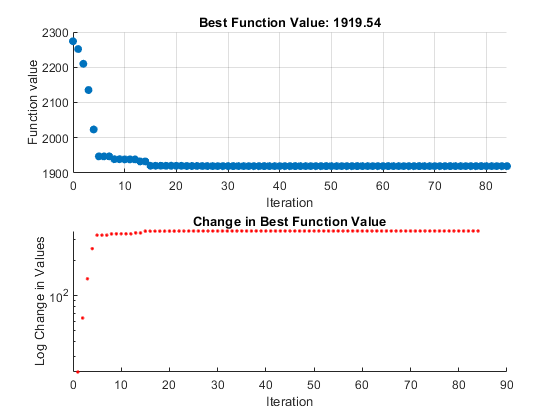Documentation

This is machine translation

Mouseover text to see original. Click the button below to return to the English version of the page.

Custom Plot Function

To use a plot function other than those included with the software, you can write your own custom plot function that is called at each iteration of the pattern search to create the plot. This example shows how to create a plot function that displays the logarithmic change in the best objective function value from the previous iteration to the current iteration. More plot function details are available in Plot Options.

Creating the Custom Plot Function

To create the plot function for this example, copy and paste the following code into a new function file in the MATLAB® Editor:

```function stop = psplotchange(optimvalues, flag) % PSPLOTCHANGE Plots the change in the best objective function % value from the previous iteration. % Best objective function value in the previous iteration persistent last_best stop = false; if(strcmp(flag,'init')) set(gca,'Yscale','log'); %Set up the plot hold on; xlabel('Iteration'); ylabel('Log Change in Values'); title(['Change in Best Function Value']); end % Best objective function value in the current iteration best = min(optimvalues.fval); % Set last_best to best if optimvalues.iteration == 0 last_best = best; else %Change in objective function value change = last_best - best; plot(optimvalues.iteration, change, '.r'); end ```

Then save the file as `psplotchange.m` in a folder on the MATLAB path.

Setting Up the Problem

The problem is the same as Linearly Constrained Problem. To set up the problem:

1. Enter the following at the MATLAB command line:

```x0 = [2 1 0 9 1 0]; Aineq = [-8 7 3 -4 9 0]; bineq = 7; Aeq = [7 1 8 3 3 3; 5 0 -5 1 -5 8; -2 -6 7 1 1 9; 1 -1 2 -2 3 -3]; beq = [84 62 65 1];```
2. Enter `optimtool` to open the Optimization app.

3. Choose the `patternsearch` solver.

4. Set up the problem to match the following figure.5. Since this is a linearly constrained problem, set the Poll method to `GSS Positive basis 2N`.Using the Custom Plot Function

To use the custom plot function, select Custom function in the Plot functions pane and enter `@psplotchange` in the field to the right. To compare the custom plot with the best function value plot, also select Best function value.Now, when you run the example, pattern search displays the plots shown in the following figure.Note that because the scale of the y-axis in the lower custom plot is logarithmic, the plot shows only changes that are greater than 0.

To run this problem using command-line functions:

```x0 = [2 1 0 9 1 0]; Aineq = [-8 7 3 -4 9 0]; bineq = 7; Aeq = [7 1 8 3 3 3; 5 0 -5 1 -5 8; -2 -6 7 1 1 9; 1 -1 2 -2 3 -3]; beq = [84 62 65 1]; options = optimoptions('patternsearch',... 'PlotFcn',{@psplotbestf,@psplotchange},... 'PollMethod','GSSPositiveBasis2N'); [x,fval] = patternsearch(@lincontest7,x0,... Aineq,bineq,Aeq,beq,[],[],[],options);```

How the Plot Function Works

The plot function uses information contained in the following structures, which the Optimization app passes to the function as input arguments:

• `optimvalues` — Current state of the solver, a structure

• `flag` — Current status of the algorithm, a character vector

The most important statements of the custom plot function, `psplotchange.m`, are summarized in the following table.

Custom Plot Function Statements

StatementDescription
`persistent last_best`

Creates the persistent variable `last_best`, the best objective function value in the previous generation. Persistent variables are preserved over multiple calls to the plot function.

`set(gca,'Yscale','log')`

Sets up the plot before the algorithm starts.

`best = min(optimvalues.fval)`

Sets `best` equal to the minimum objective function value. The field `optimvalues.fval` contains the objective function value in the current iteration. The variable `best` is the minimum objective function value. For a complete description of the fields of the structure `optimvalues`, see Structure of the Plot Functions.

`change = last_best - best`

Sets the variable `change` to the best objective function value at the previous iteration minus the best objective function value in the current iteration.

`plot(optimvalues.iteration, change, '.r')`

Plots the variable `change` at the current objective function value, for the current iteration contained in`optimvalues.iteration`.

Watch now